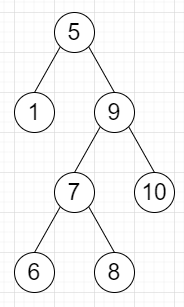# Program to find the maximum width of a binary tree in Python

Suppose we have a binary tree, we have to find the maximum width of any level in the tree. Here the width of a level is the number of nodes that can hold between the leftmost node and the rightmost node.

So, if the input is likethen the output will be 2

To solve this, we will follow these steps−

• create a map d, to hold minimum and maximum values, minimum is initially infinity and maximum is 0

• Define a function dfs() . This will take root, pos := 0, depth := 0

• if root is null, then o return

• d[depth, 0] = minimum of d[depth,0] and pos

• d[depth, 1] = maximum of d[depth,1] and pos

• dfs(left of node, 2*pos, depth+1)

• dfs(right of node, 2*pos+1, depth+1)

• From the main method, do the following−

• dfs(root)

• mx:= 0

• for each min-max pairs in list of all values of d, do

• left := min, right := max

• mx:= maximum of mx, right-lelf+1

• return mx

Let us see the following implementation to get better understanding −

## Example

Live Demo

from collections import defaultdict
class TreeNode:
def __init__(self, data, left = None, right = None):
self.data = data
self.left = left
self.right = right
class Solution:
def solve(self, root):
d=defaultdict(lambda: [1e9,0])
def dfs(node, pos=0, depth=0):
if not node:
return
d[depth]=min(d[depth],pos)
d[depth]=max(d[depth],pos)
dfs(node.left,2*pos,depth+1)
dfs(node.right,2*pos+1,depth+1)
dfs(root)
mx=0
for interval in d.values():
l,r=interval
mx=max(mx,r-l+1)
return mx

ob = Solution()
root = TreeNode(5)
root.left = TreeNode(1)
root.right = TreeNode(9)
root.right.left = TreeNode(7)
root.right.right = TreeNode(10)
root.right.left.left = TreeNode(6)
root.right.left.right = TreeNode(8)
print(ob.solve(root))

## Input

root = TreeNode(5)
root.left = TreeNode(1)
root.right = TreeNode(9)
root.right.left = TreeNode(7)
root.right.right = TreeNode(10)
root.right.left.left = TreeNode(6)
root.right.left.right = TreeNode(8)

## Output

2